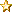###Author Topic: Maths question: How many possible setups...?  (Read 250 times)

#### joejoyce

• Newbie
•• Posts: 38
• Location: New York##### Re: Maths question: How many possible setups...?
« on: September 29, 2018, 09:58:55 pm »
Let's start with rooks. How many rook combinations are there? There are 2 rooks, each of which can be 18 similar but not identical pieces. SO there are 18x18=324 possible combinations for rooks. This number will be the same for the knight and bishop pairs, so that will give you 324x324x324 possible combos for the B, N, R pieces. Since the king is always just a standard king, it's multiplicative value is 1, and the queen's is 18. So the back rank is 324x324x324x1x18.

Okay, now the pawns; each pair of pawns gives us 324 possibilities, and there are 4 pairs, giving us 324x324x324x324, which will multiply with the back rank to give the answer. Since so far I'm doing this in my head, I'm keeping the numbers simple... 324x324=90000+12000+2400 (300x300+2x20x300+2x4x300) = 104.400, using European notation, or 104,400 for US notation.

Now I'm gonna cheat! call 104400 ~= 100000, so the pawns have ~100000x100000=10000000000 possibilities, roughly (it's a little low). Now, the back rank is 324x324x324x18, or 100000x324x18 ~= 100000x300x20=600000000, giving a final estimate of  10000000000x600000000=6000000000000000000, or 6x10^18, and this is a little low.

Grin, somebody want to check my numbers? It's been a long time since I did probabilities!